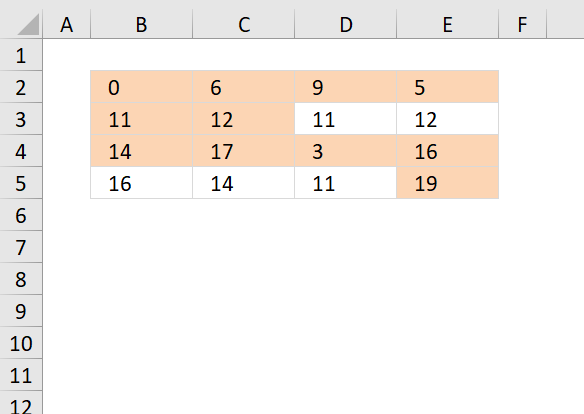Author: Oscar Cronquist Article last updated on November 22, 2018The following formula highlights cells that contain unique distinct values, in other words, all duplicate values except the first instance are not highlighted.

Conditional formatting formula:

=IF(COLUMNS(\$A\$1:A1)=1, COUNTIF(\$B\$2:B2, B2), COUNTIF(\$B\$2:B2, B2)+COUNTIF(OFFSET(\$B\$2:\$E\$5, , , 4, COLUMNS(\$A\$1:A1)-1), B2))=1

### How to apply conditional formatting

2. Go to "Home" tab on the ribbon.
3. Press with left mouse button on "Conditional formatting" button.
4. Press with left mouse button on "New Rule.."
5. Press with left mouse button on "Use a formula to determine what cells to format"
6. Copy the conditional formatting formula above and paste to "Format values where this formula is true:"
7. Press with left mouse button on Format button
8. Select a formatting.
9. Press with left mouse button on OK button.
10. Press with left mouse button on OK button.

### Explaining CF formula in cell B2

There are two parts in this formula, one part determines if a value is a duplicate in the first column. The second part of the formula determines if a value is a duplicate in the remaining columns.

The reason the formula looks like this is because of the order of how Excel calculates cells.

IF(logical_expression, first_part, second_part)

#### Step 1 - Check if first column is being evaluated

The COLUMNS function counts columns in a cell reference. \$A\$1:A1 is an expanding cell reference, it grows because A1 is a relative cell reference that changes between cells.

COLUMNS(\$A\$1:A1)=1

becomes

1=1 and returns TRUE.

#### Step 2 - Count cells based on a condition

The IF function changes the calculation based on the logical expression in the first argument. The second argument is calculated if the logical expression returns TRUE, the third argument is calculated if the logical expression returns FALSE.

The COUNTIF function makes sure that duplicates are not highlighted, only the first instance of each value. However this works only in the first column, the remaining columns need a different formula in order to do correct calculations.

IF(COLUMNS(\$A\$1:A1)=1,COUNTIF(\$B\$2:B2,B2),COUNTIF(\$B\$2:B2,B2)+COUNTIF(OFFSET(\$B\$2:\$E\$5,,,4,COLUMNS(\$A\$1:A1)-1),B2))=1

becomes

IF(TRUE,COUNTIF(\$B\$2:B2,B2),...)=1

becomes

IF(TRUE,COUNTIF(0,0),...)=1

becomes

1=1

and returns TRUE. Cell B2 is highlighted.

#### Step 3 - Calculations in remaining columns

If we move to cell C2 the IF function behaves differently.

IF(COLUMNS(\$A\$1:A1)=1, COUNTIF(\$B\$2:C2, C2), COUNTIF(\$B\$2:C2, C2)+COUNTIF(OFFSET(\$B\$2:\$E\$5, , , 4, COLUMNS(\$A\$1:B1)-1), C2))=1

becomes

IF(COLUMNS(\$A\$1:B1)=1, COUNTIF(\$B\$2:C2, C2),COUNTIF(\$B\$2:C2, C2)+COUNTIF(OFFSET(\$B\$2:\$E\$5, , , 4, COLUMNS(\$A\$1:B1)-1), C2))=1

becomes

IF(2=1, COUNTIF(\$B\$2:C2, C2), COUNTIF(\$B\$2:C2, C2)+COUNTIF(OFFSET(\$B\$2:\$E\$5, , , 4, COLUMNS(\$A\$1:B1)-1), C2))=1

becomes

IF(FALSE, COUNTIF(\$B\$2:C2, C2), COUNTIF(\$B\$2:C2, C2)+COUNTIF(OFFSET(\$B\$2:\$E\$5, , , 4, COLUMNS(\$A\$1:B1)-1), C2))=1

becomes

IF(FALSE, COUNTIF(\$B\$2:C2, C2), COUNTIF({0,6}, 6)+COUNTIF(OFFSET(\$B\$2:\$E\$5, , , 4, COLUMNS(\$A\$1:B1)-1), C2))=1

The OFFSET function returns an expanding cell reference that grows as the CF moves from column to column.

IF(FALSE, COUNTIF(\$B\$2:C2, C2), 1+COUNTIF(OFFSET(\$B\$2:\$E\$5, , , 4, 1), C2))=1

becomes

IF(FALSE, COUNTIF(\$B\$2:C2, C2), 1+COUNTIF(\$B\$2:\$B\$5, C2))=1

becomes

IF(FALSE, COUNTIF(\$B\$2:C2, C2), 1+COUNTIF({0;11;14;16},6))=1

becomes

IF(FALSE, COUNTIF(\$B\$2:C2, C2), 1+0)=1

becomes

1=1 and returns TRUE. Cell C2 is highlighted.

### Get Excel file

highlight unique distinct values in a range.xlsx